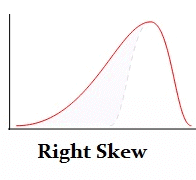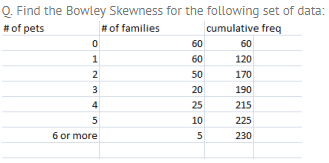Bowley’s Coefficient of Skewness, Business Mathematics & Statistics

# Bowley’s Coefficient of Skewness, Business Mathematics & Statistics - Business Mathematics and Statistics - B Com

## What is Bowley Skewness?

Bowley skewness is a way to figure out if you have a positively-skewed or negatively skewed distribution. One of the most popular ways to find skewness is the Pearson Mode Skewness formula. However, in order to use it you must know the mean, mode (or median) and standard deviation for your data. Sometimes you might not have that information; Instead you might have information about your quartiles. If that’s the case, you can use Bowley Skewness as an alternative to find out more about the asymmetry of your distribution. It’s very useful if you have extreme data values (outliers)or if you have an open-ended distribution.## Bowley Skewness Worked ExampleStep 1: Find the Quartiles for the data set. You’ll want to look for the “nth” observation using the following formulas:
Q1 = (total cum freq + 1 / 4)th observation = (230 + 1 / 4 ) = 57.75
Q2 = (total cum freq + 1 / 2)th observation = (230 + 1 / 2 ) = 115.5
Q3 = 3 (total cum freq + 1 / 4)th observation = 3(230 + 1 / 4) = 173.25

Step 2: Look in your table to find the nth observations you calculated in Step 1:
Q1 = 57.75th observation = 0
Q2 = 115.5th observation = 1
Q3 = 173.25th observation = 3

Step 3: Plug the above values into the formula:
Skq = Q3 + Q1 – 2Q2 / Q3 – Q1
Skq = 3 + 0 – 2 / 3 – 0 = 1/3

Skq = + 1/3, so the distribution is positively skewed.

## Why Bowley Skewness works.

In a symmetric distribution, like the normal distribution, the first (Q1) and third (Q3) quartiles are at equal distances from the mean (Q2). In other words, (Q3-Q2) and (Q2-Q1) will be equal. If you have a skewed distribution then there will be a difference between those two values.

## Limitations of Bowley Skewness.

Bowley Skewness is an absolute measure of skewness. In other words, it’s going to give you a result in the units that your distribution is in. That’s compared to the Pearson Mode Skewness, which gives you results in a dimensionless unit — the standard deviation. This means that you cannot compare the skewness of different distributions with different units using Bowley Skewness.

Question for Bowley’s Coefficient of Skewness, Business Mathematics & Statistics
Try yourself:
What is Bowley Skewness?

## Alternative Bowley Skewness formula.

According to Business Statistics, Bowley recognized that the Bowley Skewness formula could not be used to compare different distributions with different units. For example, you can’t compare a distribution measured in heights in cm with one of weights in pounds. He offered an alternative formula. You should use this formula if you want to compare different distributions with different units:

Relative Skewness = (Q3 + Q1) – (2 * Median / Q3 – Q1).

If you prefer an online interactive environment to learn R and statistics, this free R Tutorial by Datacamp is a great way to get started. If you're are somewhat comfortable with R and are interested in going deeper into Statistics, try this Statistics with R track.

The document Bowley’s Coefficient of Skewness, Business Mathematics & Statistics | Business Mathematics and Statistics - B Com is a part of the B Com Course Business Mathematics and Statistics.
All you need of B Com at this link: B Com

115 videos|142 docs

## FAQs on Bowley’s Coefficient of Skewness, Business Mathematics & Statistics - Business Mathematics and Statistics - B Com

 1. What is Bowley's Coefficient of Skewness?Ans. Bowley's Coefficient of Skewness is a measure of the degree and direction of skewness in a given data set. It is also known as quartile skewness coefficient or Bowley's skewness coefficient. It is calculated using the formula (Q3 + Q1 – 2Q2) / (Q3 – Q1), where Q1, Q2, and Q3 are the first, second, and third quartiles, respectively. The resulting value ranges from -1 to +1, where a negative value indicates a negative skewness, a positive value indicates a positive skewness, and a value of zero indicates a symmetric distribution.
 2. How is Bowley's Coefficient of Skewness useful in business and statistics?Ans. Bowley's Coefficient of Skewness is a useful tool in business and statistics as it helps in determining the shape of a distribution. It is used to provide information about the degree and direction of skewness in a given data set. This information is important in making decisions in areas such as finance, marketing, and economics. For example, if a distribution is positively skewed, it may indicate a potential problem with a marketing campaign or pricing strategy.
 3. How does Bowley's Coefficient of Skewness differ from other measures of skewness?Ans. Bowley's Coefficient of Skewness differs from other measures of skewness, such as Pearson's skewness coefficient and the moment coefficient of skewness, in that it is based on quartiles rather than on the mean and standard deviation. This makes it less sensitive to outliers than other measures of skewness. Additionally, it can be used for both continuous and discrete data sets.
 4. What are some limitations of using Bowley's Coefficient of Skewness?Ans. One limitation of using Bowley's Coefficient of Skewness is that it only provides information about the degree and direction of skewness and does not provide any information about the shape of the distribution. Additionally, it may not always be appropriate to use Bowley's Coefficient of Skewness, as it assumes that the data set is unimodal, that is, it has only one mode. If the data set has multiple modes, Bowley's Coefficient of Skewness may not accurately represent the degree and direction of skewness.
 5. What are some real-life applications of Bowley's Coefficient of Skewness?Ans. Bowley's Coefficient of Skewness has several real-life applications. For example, it can be used in finance to determine the skewness of a portfolio's returns. A positively skewed portfolio may indicate that the portfolio has a higher potential for gains, but also a higher potential for losses. In marketing, Bowley's Coefficient of Skewness can be used to analyze customer satisfaction surveys, with a positively skewed distribution indicating an area where improvements can be made. In economics, Bowley's Coefficient of Skewness can be used to analyze income distributions, with a positively skewed distribution indicating a greater concentration of wealth in a few individuals.

115 videos|142 docsExplore Courses for B Com examSignup to see your scores go up within 7 days! Learn & Practice with 1000+ FREE Notes, Videos & Tests.
10M+ students study on EduRev
Track your progress, build streaks, highlight & save important lessons and more!
Related Searches

,

,

,

,

,

,

,

,

,

,

,

,

,

,

,

,

,

,

,

,

,

,

,

,

;